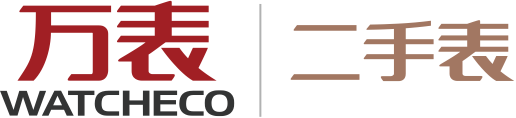| 购物车()

#1000以下 1000-3000 3000-5000 5000-10,000 10,000-50,000 50,000-100,000 100,000以上

• 浪琴
• 欧米茄
• 天梭
• 劳力士
• 美度
• 爱宝时
• 帝舵
• 艾美
• 阿玛尼
• 泰格豪雅
• 豪利时
• 康斯登
• 其他手表
• 上海
• 万国
• 卡地亚
• 西铁城
• 乔治菲登
• 宝齐莱
• 雷达
• CIGA Design
• 迪沃斯
• 赫柏林
• 梅花
• 拓天马
• 百年灵
• 卡西欧
• 万宝龙
• SevenFriday
• 柏高
• 格拉苏蒂∙原创
• 积家
• 索鱼
• 汉米尔顿
• 依波路
• 盖尔斯
• 海鸥
• 伯爵
• 古驰
• 北京
• 艾美达
• 英纳格
• 江诗丹顿
• 瑞士军表Swiss Military
• 特工
• 优立时
• 百达翡丽
• 宝珀
• 精工
• 宝格丽
• 名士
• 雅典
• 瑞宝
• 芝柏
• 摩凡陀
• 爱马仕
• 雍加毕索
• 梵德宝
• 昆仑
• 东方双狮
• 库尔沃
• 雅克利曼
• 爱彼
• CK
• 萧邦
• 飞亚达
• 宇舶
• DW丹尼尔·惠灵顿
• 波士
• 蕾蒙威
• 沛纳海
• 查梅斯
• 威廉
• 法穆兰
• 莫勒
• 诺美纳
• 驰客
• 爱罗
• 艾米龙
• 天王
• 艾沛勒
• 华为
• 万希泉
• 尚维沙
• 佳明
• 罗贝罗班
• 雷米格
• 朗格
• 罗蒂诗蔓
• LACOSTE
• DVH
• 帕玛强尼
• 孔雀
• 天铭
• 恒圆
• 雅克德罗
• 迪奥
• 浪陀
• 路易威登
• 冠蓝狮（大精工）
• 真力时
• 宝玑
• 罗杰杜彼
• 人群
男表 女表 中性
机芯
石英机芯 机械机芯 电子机芯
抱歉，没有找到相关商品，为您推荐以下商品
上一页 下一页